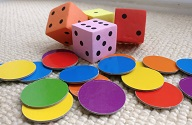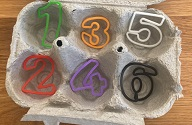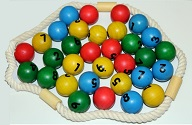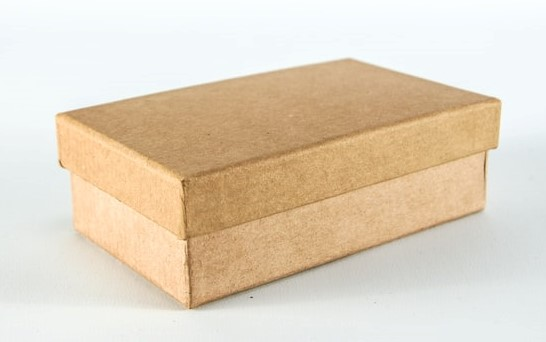# The Box Game

##### Age 3 to 5
Solving problemsChildren often enjoy visualising how many toys are hidden in a box.

Adults could start by using three large toys and a giant box with a group of young children. Then use bigger numbers or miniature toys with smaller groups or individuals.

The Activity
Put toys one at a time into the box, so children cannot see them inside, counting all together. Ask: "Can you show on your fingers how many are hidden?" Display a large numeral.
Add one to the box, without showing the objects inside, and ask children to show on their fingers, "How many are there now?". Then show how many are inside the box and count to check.

Encouraging mathematical thinking and reasoning:

Describing
How many were there at the start?
How many are there now?

Reasoning
How do you know?
How did you work it out?

Opening Out
What if we add two more?
What if we take one out? Two out?
Imagine there are 10 in there and I take out 6 - how would you know how many were left?

Recording
Can you show how many there were?
Can you show how many there are now?
Can you show how many there were and what happened?

The Mathematical Journey

Counting and cardinality:

• saying numbers in the right order
• saying one number for each object
• saying how many there are by showing 'finger numbers', by counting fingers, and instantly (without counting)

Matching numerals and amounts:

• selecting numerals to match the starting and finishing numbers

• predicting adding one to a number (or two)
• modelling on fingers
• counting all e.g. putting up four, then two more, then counting from one through to six
• counting on, starting from the first number - "Four, five, six"
• visualising or counting mentally, e.g. nodding at hidden objects or saying "I went four, five, six"
• using number facts - "Because there were three and you put one more", "I know two and two is four"

Subtracting:

• predicting taking one from a number (or two)
• modelling on fingers
• counting all, then how many are left - putting up six, putting down two, counting the four still up
• visualising or counting backwards mentally - "I went six, five, four so there are four"

Development and Variation
Increase numbers to start, to add and take away:
• Repeatedly add one to the previous number.
• Repeatedly subtract one from the previous number.
• Vary the starting number, but just add one each time (or two).
• Keep one starting number and subtract varying amounts to build number fact knowledge.
• Repeatedly add two or subtract two from a starting number.
Children can choose how many to add or take away.

Vary the context: use pennies in a pot, children behind a screen, dinosaurs in a cave. Model with fingers, Numicon, large number line, 'staircase' of cubes or other resources.

ResourcesBox or tin with lid, a pot to upturn or a screen, cloth, cave...
Toys, pennies, children, dinosaurs...
Large numerals to display.